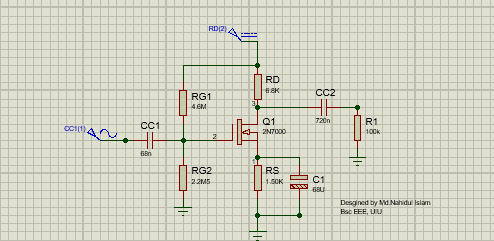Design an NMOS amplifier with following specifications. Given specs: AV = 50 and VDD = 12 V, Rin is of MΩ order.

Single stage common source amplifier

In electronics, a common-source amplifier is one of three basic single-stage field-effect transistor (FET) amplifier topologies, typically used as a voltage or transconductance amplifier. The remaining terminal is what is known as "common". In this example, the signal enters the gate, and exits the drain. The only terminal remaining is the source. This is a common-source FET circuit. As a voltage amplifier, input voltage modulates the current flowing through the FET, changing the voltage across the output resistance according to Ohm's law. However, the FET device's output resistance typically is not high enough for a reasonable. The common source circuit provides a medium input and output impedance levels. Both current and voltage gain can be described as medium, but the output is the inverse of the input, i.e. 180° phase change. This provides a good overall performance and as such it is often thought of as the most widely used configuration.

## Design process of an NMOS amplifier

NMOS amplifier with following specifications

AV = 50
VDD = 12 V
Rin is of MΩ order
2N7000 MOSFET
lowest frequency (20 Hz)

#### Step 1 : kn calculation

From Datasheet of 2N7000, VGS,off = 2 V (VTh) , VGS,on = 10 V and ID,on = 1 A

Then, 𝑘𝑛 = (2𝐼𝐷,𝑜𝑛) /(𝑉𝐺𝑆𝑜𝑛−𝑉𝑇ℎ)^2 gives kn = (2 * 1 A)/(10 -2)^2 = 0.03125 A/V^2

#### Step 2: Q-point calculation

𝑉𝐺𝑆𝑞 = (𝑉𝐷𝐷/𝐴𝑉)+ 𝑉𝑇ℎ = 12/50 + 2 = 2.24 V

𝐼𝐷𝑞=12𝑘'𝑛(𝑉𝐺𝑆𝑞−𝑉𝑇ℎ)2= 0.5 *0.03125 *(2.24 – 2)^2 = 0.0009A

#### Step 3: Choosing Bias resistors

𝑅𝐷=(0.5*𝑉𝐷𝐷)/𝐼𝐷𝑞 = 0.5 * 12 / 0.0009 = 6.67 KΩ. I choose RD as 6.8 KΩ (standard value)

𝑅𝑆=𝑅𝐷/5 = 6.67/5 = 1.35 KΩ. I choose RS as 1.5 KΩ (standard value)

Rin is of MΩ and Rin = R1 || R2 and R1 > R2,

Let R2 = 2.2 MΩ.

𝑉𝐺=𝑉𝐺𝑆𝑞+ 𝑉𝑆=𝑉𝐺𝑆𝑞+𝐼𝐷𝑞𝑅𝑆=𝑅2𝑅1+ 𝑅2 ×𝑉𝐷𝐷

2.24 V + 0.0009 A * 1.5 K = 2.2 𝑀𝑅1+ 2.2 𝑀 ×12

2.24 + 1.35 = 2.2 𝑀𝑅1+ 2.2 𝑀 ×12

R1 = 4. 68 MΩ.

I choose R1 as 4.7 MΩ (standard value)

#### Step 4: Choosing Capacitors for fmin = 20 Hz

𝐶c1 ≥1/(0.2𝜋 ∗𝑓𝑚𝑖𝑛 ∗ 𝑅𝑇𝐻)=1/0.2𝜋 ∗𝑓𝑚𝑖𝑛 ∗ (𝑅1 || 𝑅2)

Cc1 ≥1/(0.2𝜋 ∗20 ∗ (4.7∗2.2)/(4.7+2.2) M)

Cc1 ≥ 53.13 nF

I choose CC1 as 68 nF (standard value)

𝐶c2 ≥1/{0.2𝜋 ∗𝑓𝑚𝑖𝑛 ∗ 𝑅𝑇𝐻)=1/(0.2𝜋 ∗𝑓𝑚𝑖𝑛 ∗ (𝑅𝐷+𝑅𝐿)}

Cc2 ≥1/{0.2𝜋 ∗20 ∗ (6.8 + 100) 𝐾}

Cc2 ≥ 745.49 nF

I choose Cc2 as 820 nF (standard value)

𝐶𝑠 ≥1/{0.2𝜋 ∗𝑓𝑚𝑖𝑛 ∗ 𝑅𝑇𝐻=10.2𝜋 ∗𝑓𝑚𝑖𝑛 ∗ 𝑅𝑆}

𝐶s ≥1/{0.2𝜋 ∗20 ∗ 1.5 𝐾}

Cs ≥ 53078.56 nF = 53.078 uF

I choose Cs as 68 uF (standard value)

#### Component

2N7000 type MOSFET
12v Supply
RG1 = 4.68M
𝑅𝐷 = 6.8k
Rs = 1.5k
RG2 =2.2M
Cc1 = 68nF
Cc2 = 820nF
Cs = 68uFDesigned by Md.Nahidul Islam